#### The deep dive into the world of model assessment metrics that you didn’t know you needed to know — until now

The code used to generate the graphs and the KS Area Between Curves computation in this post is available as part of the dython library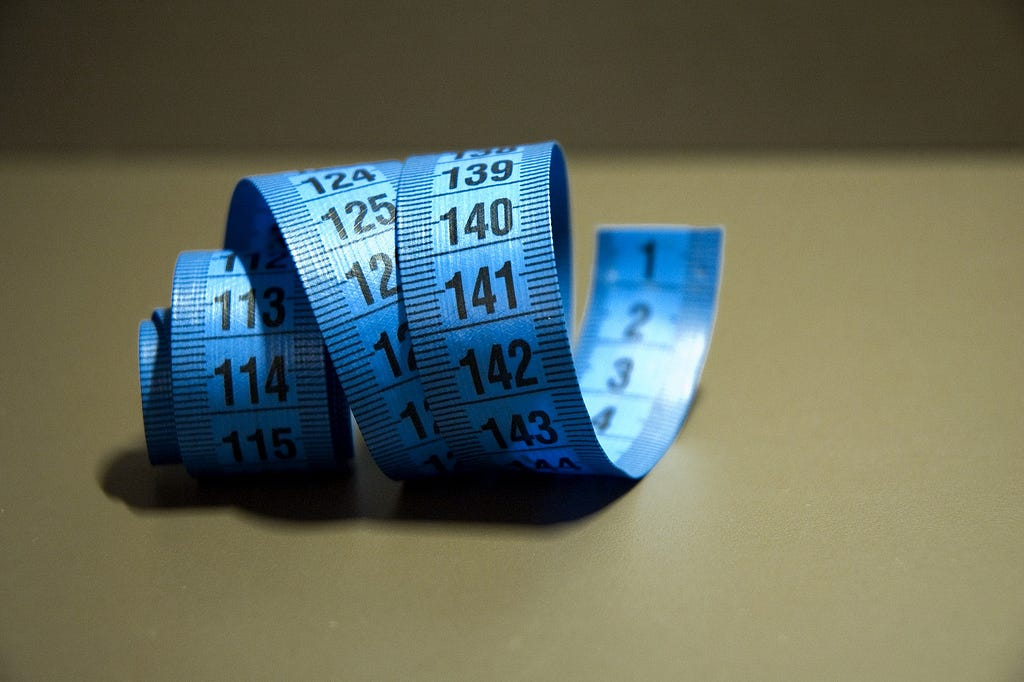Image by lloorraa from Pixabay

Here’s a classic scenario you probably ran into as a Data Scientist — you need to train a binary classifier, say a simple logistic regression, over a rather small and imbalanced data — for example: CTR prediction (Click-Through Rate, meaning — will a user click on an ad or not). So again, you don’t have a lot of data, and it’s highly imbalanced, somewhere around the negative/positive ratio of 1000:1.

Your goal, then, is to predict the probability a click will occur given a binary label — in other words, you’ll predict a continuous number, while the true labels are binary classes. Nothing uncommon — you probably already know how you’re going to implement it. But how do you intend to measure how successful your classifier is? Which metric will you use? This, as I just found out, isn’t as trivial as I thought. Let me take you through what I’ve just learnt.

### The Obvious “No”s

Whenever we need to come up with a metric, it’s our human nature to think of accuracy, which is defined by the number of overall correctly-classified samples out of the entire dataset. As our dataset is highly imbalanced, this metric is skewed towards the majority class, so even if the model predicts always 0s, the accuracy is still very high.

It’s worth mentioning here that subsampling this specific dataset to a negative/positive ratio of 1:1 is not recommended, as we’ve said the dataset is small — and that means we can’t afford to lose so much valuable data. Oversampling the positive class on the other hand is meaningless, as it does the exact opposite of what we’re trying to check — the model’s ability to generalize. So we must accept the fact that our data is skewed, and find a metric that can handle it.

Precision and Recall are also not what we’re looking for, as they only draw a partial picture, by focusing only on the positive class. While there are some use cases where this suits us well, this isn’t always the case — as in CTR prediction — where we actually care about both classes, and wish to classify them both accurately. F1 score is also a no-go, as it’s basically a mix of these two metrics.

### Area Under Curve, and Why It Doesn’t Work

There’s an implicit issue with the metrics I mentioned above that we ignored. All metrics that are based on the Confusion Matrix are intended for binary labels and outputs — meaning, the true labels are 0 and 1, and so is the output. But our model predicts probability, not a binary class. In order to transform probability to a binary class, we need to decide on a threshold, where all probabilities higher than it will be classified as 1, and the rest 0. The go-to threshold is 0.5, but that’s not necessarily the best choice (if you’re not sure why, check this blogpost I wrote). This means that the above metrics are not only skewed towards the majority class, they can also be biased by our decision of threshold. Bad.

This is why one of the most common metrics in the ML world is ROC AUC (Receiver Operating Characteristic Area Under Curve). I’m assuming you’re familiar with it, but in case you’re not — check the link at the paragraph above. Now, the ROC curve helps us find the optimal threshold to convert probabilities to a binary class. But wait, we said we want to predict probabilities, why do we need a metric that helps us find a way to convert it to binary labels?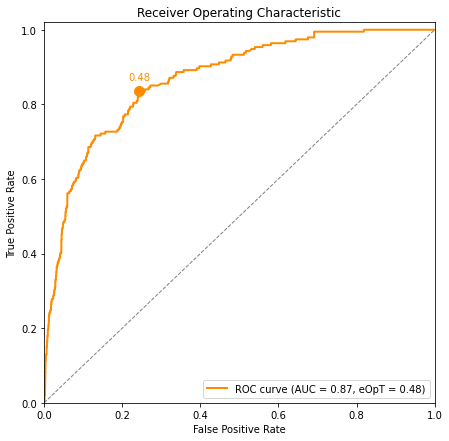A ROC curve. The dashed line marks the curve of a naive classifier, and eOpT marks the estimated optimal threshold

The answer is we don’t. But there’s another thing we can learn from the ROC curve — separation. You see, the better the model is — the more the positive samples will revolve around a probability of 1, and negative samples will be closer to 0. So the better the model is, the better it is at separating the positive and negative probability distributions from one another. And we can actually see this in the ROC curve — the better the separation, the larger the area under the curve is. So we can use the area under the curve, or AUC, as a metric to help us assess the model. Great! — But not really.

As this blogpost by Jason Brownlee clearly explains, ROC curves are actually affected by the data imbalance too, as the metrics on both axes are mixing the two classes. So instead, he suggests to use another curve: the Precision-Recall curve, where we draw the Precision over the y-axis and the Recall over the x-axis for all thresholds.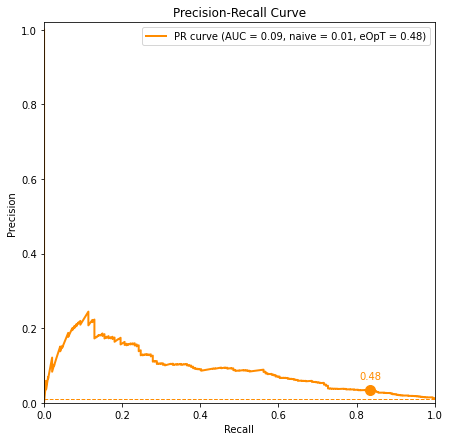A PR curve. The dashed line marks the curve of a naive (untrained) model

Wait a minute. A few paragraphs ago I explained why these two metrics are not useful in our case. Did I change my mind? No. I’m not suggesting to use these two metrics as they are, but to use the curve they create. Because, just like in the ROC curve, the AUC of this curve is a measure of the model’s class separation — the higher the better, with a maximal value of 1. So we can use the PR AUC instead? Well, yes — but there’s an issue.

When considering the ROC AUC, we know for sure that a naive classifier, meaning a classifier that didn’t learn a thing, will always have an AUC = 0.5. This means we have a fixed baseline, and this allows us to keep track of a model’s ROC AUC score over time, as it trains periodically on different datasets. As long as all datasets come from similar distributions, a model’s ROC AUC score should be more or less the same.

But with PR AUC, things work a little differently, as a naive model’s Precision-Recall curve is a flat horizontal line of some fixed precision. What is that value? It’s the fraction of positive samples out of the entire dataset. And that means that the baseline to which we compare our model to isn’t fixed, and depends on the dataset we use. If we always use the same test-set, this shouldn’t be a problem, but that’s never the case in real-life situations.

How can we tackle that? Well, one way is to use the ratio between the model’s PR AUC and a naive model’s PR AUC (tested both on exactly the same data) as a metric, but this can become too volatile and hard to use for comparisons over time. So while it is possible to use it, I believe we all want a metric which isn’t that noisy.

### Comparing Distributions

The keyword of the last section is separation. Think about it — what we really are after here is class separation — or in other words, we’re looking for a model which predicts two completely different probability distributions for the two classes. Well, comparing two distributions is almost a synonym to computing the KL-Divergence of these two distributions: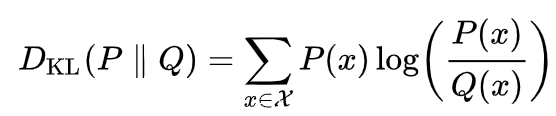Discrete KL-Divergence. Taken from Wikipedia

But, while KL-Divergence is usually a go-to metric in such cases, it actually isn’t a good metric in our case, for two reasons:

1. The input order to KL-Divergence matters. If P and N are the positive and negative probability distributions, then KL(P,N) KL(N,P). This is a recipe for disaster and wrong comparisons.
2. Technically, we don’t really know the distributions, as we only hold a list of values sampled out of these distributions. That means we’re using the discrete KL-Divergence. Why does that matters? Because we’re running over probability prediction values varying from 0 to 1. The chances of hitting an empty bucket with no samples in it are very high, especially for small datasets — and when that happens, KL-Divergence explode (see formula above whenever P or Q are 0). While we can technically work it out by adding some small-enough constant ε to P and Q, the metric becomes super-dependent in the value of that constant, and that makes it too sensitive to manual configurations.

To fix these two issues, we can use a less known distribution-comparison metric: Hellinger Distance: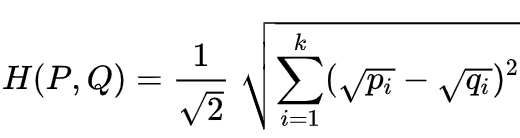Discrete Hellinger Distance. Taken from Wikipedia

The discrete case is both symmetrical and doesn’t explode when hitting empty buckets. But there’s apparently an issue with that metric too. Both distribution depicted below have the same Hellinger Distance, a perfect score of 1, which is obviously not what we want. We need something else.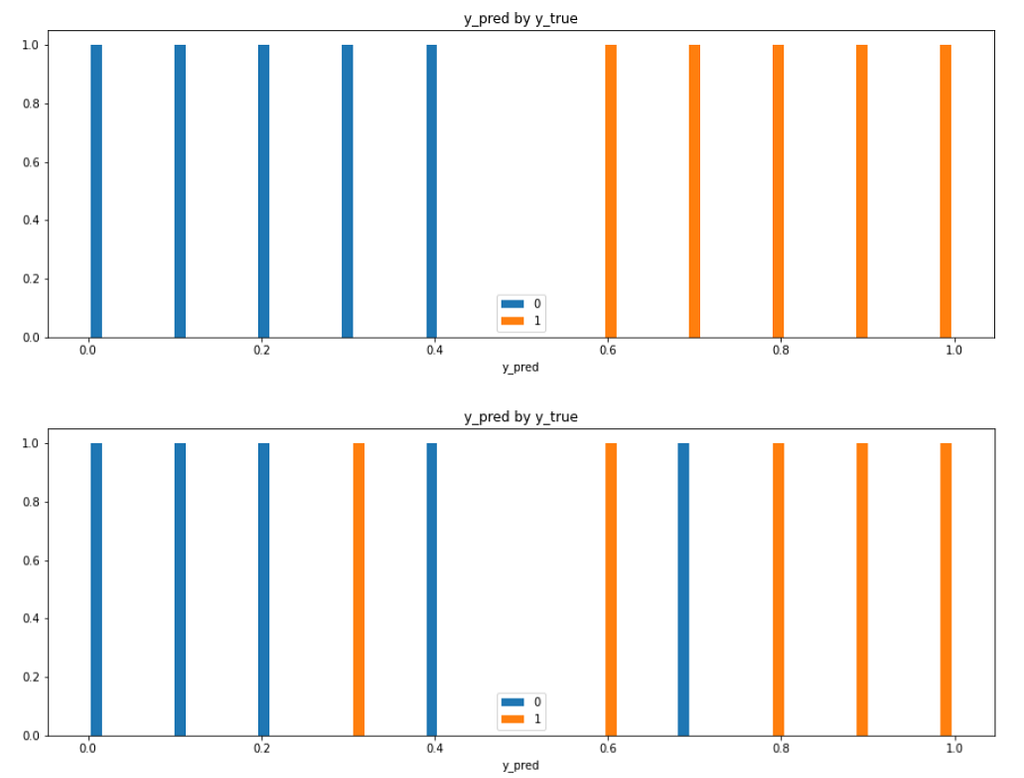Two different distributions, one with perfect separation and one without, both having the same Hellinger Distance of 1

### Separation Is All You Need?

This whole distribution comparison thing started to create a mess inside my head, so I asked myself again — what am I really trying to measure? Again, separation. And so I decided to give Correlation Ratio a chance. I briefly discussed it in The Search for Categorical Correlation, so let me skip to the bottom line of what it does: given a set of tuples made of a continuous number and a category, it answers the question: how well can we know to which category some continuous number is associated with? This is pretty much what we seek here — given a predicted probability, how likely is it to be associated with the correct class? Correlation Ratio answers just that, and so it seemed to be the perfect metric for us. Almost.

There’s another thing we care about, and we can’t get from this metric — that is how the two distributions are distributed, not just how well they are separated. At one of the first paragraphs I mentioned that the optimal model will have two separate distributions, with the center-of-mass of the positive and negative distributions located as close as possible to 1 and 0, respectively. In other words, we’re not looking only for separation, but also for calibration — meaning, how close are the predictions to the actual labels. This piece of information is important, as we can see in the plots below — yet Correlation Ratio, as well as all other metrics we’ve encountered so far, doesn’t take this into account.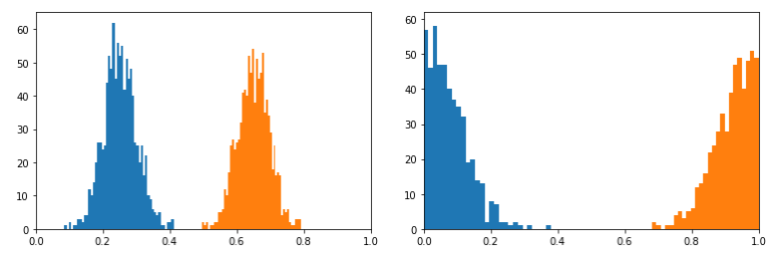Two sets of perfectly separated positive and negative distributions. We would prefer the model on the right, as the centers of mass are 0 and 1 (and even though the variances are double than the ones of the model on the left). Still, the correlation ratios of the left and the right models are 0.97 and 0.98, receptively — so it isn’t a very useful metric in cases such as this

It turned out the metric I was really after was something I had to create myself on top of another distribution-comparison test, known as the Kolmogorov–Smirnov Test. I’ll skip the general use of the KS Test and focus on how we can use it to estimate a binary classifier. Unlike all other curves we met so far, for the KS Test we draw two curves on the same plot. For each possible threshold (just like in ROC), we draw the fraction of positive examples classified correctly below that threshold, out of the total number of positive examples. We then do the same thing, but for the negative examples. We get a plot that looks like this: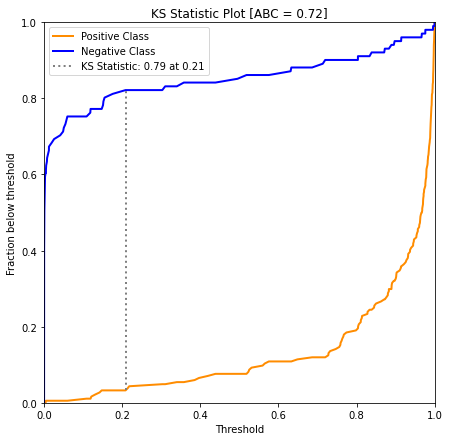KS Test curves of the positive and negative ratios under the threshold. The estimated optimal threshold is plotted as a grey vertical line

The plot above tells us that, for example, for the threshold 0.8, about 20% of positive examples received predictions lower than the threshold, and about 90% of negative examples are found below that threshold too. Like ROC curves, KS curves also allow us to find the optimal threshold for separation (depicted by the vertical line), but unlike ROC curves, KS Test is resilient to data imbalance due to the fact that it separates the positive and negative examples to two different — and normalized — curves.

This looks like another metric to optimize thresholds, but there’s something else hidden here. Consider a perfect classifier — one that predicts probability of exactly 1 when the label is 1, and exactly 0 when the label is 0. How would these curves look like? You got it right — the positive curve will drop immediately to 0, and stay there (as for any threshold lower than exactly 1, there will be no positive samples). The negative curve on the other hand, will linger constantly at 1 (as for any threshold higher than 0, all negative samples will be found). This will create a perfect square between the two curves, and any deviation from this perfect classifier will narrow down the area locked between the curves. And so, the KS Area Between Curves is the metric we seek, with an optimal value of 1 for the perfect classifier, and lower values for anything else. YES! Here’s the metric we needed so badly, and it even has a super-cool acronym: ABC. Perfect!

Want to use it yourself? It’s as simple as:

`from dython.model_utils import ks_abcks_abc(y_true, y_pred)`

Hooray!

### One More Metric Before You Go

While the KS ABC handles calibration in our specific case (meaning, in the case when the true labels are found on the extreme two edges of the scale), in the more general case we’ll need another metric for calibration. I ran into this metric which I personally liked, and so thought I’ll mention it here. It’s called the Brier Score and it looks like this: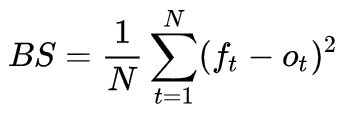Brier Score, where f marks the predictions (forecast) and o marks the true labels (outcome). Taken from Wikipedia

It’s similarity to MSE makes it very intuitive, and it automatically penalizes more heavily very off predictions rather than close ones, due to its squaring-nature. If the dataset is imbalanced, you’ll probably want to use a weighted Brier Score, giving more emphasis to the minority class. This is can also be done with 4 lines of code:

`from sklearn.metrics import brier_score_losspositive_weight = (len(y_true) - sum(y_true)) / sum(y_true)sample_weight = [positive_weight if i == 1 else 1 for i in y_true]brier_score_loss(y_true, y_pred, sample_weight=sample_weight)`

And that’s it.

### Final Words

We sometimes tend to overlook, or simply not invest enough thought into how we measure our models. Just like each problem in the ML world requires different approaches and algorithms, metrics are just the same — they must match the data and the model, as well as what it is you are really seeking the model to achieve. Not choosing the right metric can blindfold you, making you unaware of issues in the model, or alerting you over noise. I can only hope you found this post useful to you, by any scale or metric you decide to measure it :-)The Metric System: How to Correctly Measure Your Model was originally published in Towards Data Science on Medium, where people are continuing the conversation by highlighting and responding to this story.